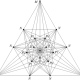Posted by: Alexandre Borovik | April 15, 2018

## If a quantity is not assigned a concrete numerical value, it does not exist for students

I ses this example in my lectures when I explain the difference between arithmetic and harmonic means:

A car traveled from A to B with speed 40 miles per hour, and back from B to A with speed 60 miles per hour. What was the average speed of the car on the round trip?

Anatoly Vorobey and Vladimir Kramchatkin made a useful comment on Facebook on this quite standard and well-known problem:

“The answer is obviously 48 [miles per hour]. 95% can not solve this problem the first time. But if they are told in advance that the distance between A and B is 120 [miles], 95% of schoolchildren will easily solve this problem.”

A concrete number, 120 km, serves as a strong hint that students are expected to do something with this number. But, for majority of students, if a magnitude or a quantity is not assigned a concrete numerical value, it does not exist. This is one of the flaws of mathematics education at schools: no-one tells students that they have to be able to see hidden parameters in arithmetic problems. But this is not the only flaw: students are also not told how to check solutions. Checking answers frequently benefits from seeing a problem in a wider context and varying the data. The standard answer that students give to the problem with the car is 50 miles per hour, the arithmetic mean of the two speeds. But this solution immediately collapses if we slightly change the problem: what would happen if the speed of the car on its way back from B to A was 0 miles per hour?

## Responses

1.For a better analysis of this situation, what would be the percentage of students able to solve it, if you say i) before or ii) after the question, that the distance between A and B is to be named L without giving a concrete value ?